## RS Aggarwal Class 7 Solutions Chapter 20 Mensuration Ex 20B

These Solutions are part of RS Aggarwal Solutions Class 7. Here we have given RS Aggarwal Solutions Class 7 Chapter 20 Mensuration Ex 20B.

Other Exercises

Question 1.
Solution:
Outer length of plot (L) = 75 m
and breadth (B) = 60 m
Width of path inside = 2 m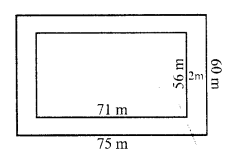Inner length (l) = 75 – 2 x 2 = 75 – 4 = 71 m
and width (b) = 60 – 2 x 2 = 60 – 4 = 56 m
Area of the path = L x B – l x b = (75 x 60 – 71 x 56) m² = 4500 – 3976 = 524 m²
Rate of constructing it = Rs. 125 per m²
Total cost = Rs. 524 x 125 = Rs. 65500

Question 2.
Solution:
Outer length of the plot (L) = 95 m
and breadth (B) = 72 m
Width of path = 3.5 mInner length (l) = 95 – 2 x 3.5 = 95 – 7 = 88 m
and breadth = 72 – 2 x 3.5 = 72 – 7 = 65 m
Outer area = L x B = 95 x 72 m² = 6840 m²
and inner area = l x b = 88 x 65 m² = 5720 m²
Area of path = outer area – inner area = 6840 – 5720 = 1120 m²
Rate of constructing it = Rs. 80 per m²
Total cost = Rs. 1120 x 80 = Rs. 89600
and rate of laying grass = Rs. 40 per m²
Total cost = Rs. 40 x 5720 = Rs. 228800
Total cost = Rs. 89600 + Rs. 228800 = Rs. 318400

Question 3.
Solution:
Length of saree (L) = 5 m
and breadth (B) = 1.3 m
Width of border = 25 cm
Inner length (l) = 5 – $$\frac { 2 x 25 }{ 100 }$$ = 5 – 0.5 = 4.5 m
and inner breadth (b) = 1.3 – $$\frac { 2 x 25 }{ 100 }$$ = 1.3 – 0.5 = 0.8 m
Now area of the boarder = L x B – l x b
= (5 x 1.3 – 4.5 x 0.8) m²
= 6.50 – 3.60
= 2.90 m²
= 2.90 x 100 x 100 = 29000 cm²
Cost of 10 cm² = Re. 1
Total cost = Rs. $$\frac { 29000 x 1 }{ 10 }$$ = Rs. 2900

Question 4.
Solution:
Inner length of lawn (l) = 38 m
and breadth (b) = 25 m.
Width of path = 2.5 m.
Outer length (L) = 38 + 2 x 2.5 = 38 + 5 = 43 m
and outer breadth (B) = 25 + 2 x 2.5 = 25 + 5 = 30 m
Area of path = Outer area – Inner area
= (43 x 30 – 38 x 25) m²
=(1290 – 950) m² = 340 m²
Rate of gravelling the path = Rs. 120 per m²
Total cost = Rs. 120 x 340 = Rs. 40800

Question 5.
Solution:
Length’ of room (l) = 9.5m
Width of outer verandah = 1.25m
Outer length (L) = 9.5 + 2 x 1.25 = 9.5 + 2.5 = 12.0 m
and breadth (B) = 6 + 2 x 1.25 = 6 + 2.5 = 8.5 m
Area of verandah = Outer area – Inner area = L x B – l x b
= (12.0 x 8.5 – 9.5 x 6) m² – (102.0 – 57.0) m² = 45 m²
Rate of cementing = Rs. 15 per m²
Total cost = Rs. 80 x 45 = Rs. 3600

Question 6.
Solution:
Each side of square bed (a) = 2m 80 cm = 2.8 m
Width of strip = 30cm
Outer side (A) = 2.8 m + 2 x 30cm = 2.8 + 0.6 = 3.4m
Outer area = (3.4 m)² = 11.56 m²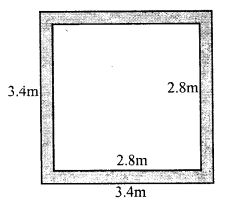Inner area = (2.8)² = 7.84 m²
Area of increased bed flower = 11.56 – 7.84 = 3.72 m²

Question 7.
Solution:
Ratio in length and breadth of the park = 2 : 1
Its perimeter = 240 m
Let length = 2x
Perimeter = 2 (2x + x) = 2 x 3x = 6x
6x = 240
⇒ x = $$\frac { 240 }{ 6 }$$ = 40
Length = 2x = 2 x 40 = 80m
and breadth = x = 40m
Area = L x B = 80 x 40 m² = 3200 m²
Width of path inside the park = 2m
Inner length (l) = 80 – 2 x 2 = 80 – 4 = 76 m
and breadth (b) = 40 – 2 x 2 = 40 – 4 = 36 m
Inner area = 76 x 36 = 2736 m²
Area of path = Outer area – Inner area = 3200 – 2736 = 464 m²
Rate of paving the path = Rs. 80 per m²
Total cost = Rs. 80 x 464 = Rs. 37120

Question 8.
Solution:
Length of hall (l) = 22m
Space left along the walls = 75m = $$\frac { 3 }{ 4 }$$ m
Inner length (l) = 22 – 2 x $$\frac { 3 }{ 4 }$$ = 20.5 m
Inner breadth (b) = 15.5 – 2 x $$\frac { 3 }{ 4 }$$ = 15.5 – 1.5 = 14m
Area of carpet = Inner area = 20.5 x 14 m² = 287 m²
Outer area = 22 x 15.5 = 341 m²
Area of strip left out = 341 – 287 = 54 m²
Width of carpet = 82 cm = $$\frac { 82 }{ 100 }$$ m
Length of carpet = 287 ÷ $$\frac { 82 }{ 100 }$$
= $$\frac { 287 x 100 }{ 82 }$$ = 350m
Rate of carpet = Rs. 60 per metre
Total cost = Rs. 60 x 350 = Rs. 21000

Question 9.
Solution:
Area of path = 165 m²
Width of path = 2.5m.
Let side of square lawn = x m.Outer side = x + 2 x 2.5 = (x + 5) m
Area of path = (x + 5)² – x²
⇒ x² + 10x + 25 – x² = 165
⇒ 10x = 165 – 25 = 140
⇒ x = $$\frac { 140 }{ 10 }$$ = 14m
Side of lawn = 14m
and area of lawn = (14)² m² = 196 m²

Question 10.
Solution:
Ratio in length and breadth of a park = 5 : 2
Width of path outside it = 2.5 m
Area of path = 305 m2
Let Inner length (l) = 5x
Outer length (L) = 5x + 2 x 2.5 = (5x + 5) m
Width (B) = 2x + 2 x 2.5 = (2x + 5) m
Area of path = Outer area – Inner area
⇒ (5x + 5) (2x + 5) – 5x x 2x = 305
⇒ 10x² + 10x + 25x + 25 – 10x² = 305
⇒ 35x = 305 – 25 = 280
⇒ x = 8
Length of park = 5x = 5 x 8 = 40m
and breadth = 2x = 2 x 8 = 16m
Dimensions of park = 40m by 16m

Question 11.
Solution:
Length of lawn (l) = 70 m
Width of crossing roads = 5m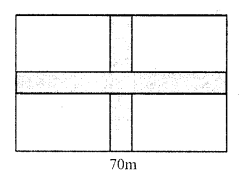Area of roads = 70 x 5 + 50 x 5 – (5)²
= 350 + 250 – (5)²
= 600 – 25 = 575 m²
Cost of constructing = Rs. 120 per m²
Total cost Rs. 120 x 575 = Rs. 69000

Question 12.
Solution:
Length of lawn (l) = 115m
Width of road parallel to length = 2m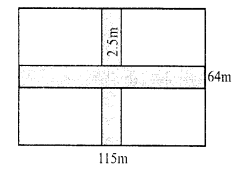Area of roads = (115 x 2 + 64 x 2.5 – 2 x 2.5) m²
= (230 + 160 – 5) m² = (390 – 5) m² = 385 m²
Cost of gravelling = Rs. 60 m²
Total cost = Rs. 60 x 385 = Rs. 23100

Question 13.
Solution:
Length of field (l) = 50 m
and breadth (b) = 40 m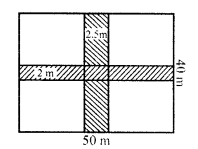Width of road parallel to length = 2 m
Area of roads = 50 x 2 + 40 x 2.5 – 2.5 x 2 = (100 + 100 – 5) m² = 195 m²
and area of remaining portion = 50 x 40 – 195 = 2000 – 195 = 1805 m²

Question 14.
Solution:
(i) Outer length = 43m
Area = 43 x 27 = 1161 m²
Inner length = 43 – 2 x 1.5 = 43 – 3 = 40m
and breadth = 27 – 2 x 1 = 27 – 2 = 25m
Inner area = 40 x 25 = 1000 m²
Area of shaded portion = 1161 – 1000 = 161 m²
(ii) Side of square (a) = 40m
Area = (a)² = 40 x 40 = 1600 m²
Area of larger road = 40 x 3 = 120 m²
and area of shorter road = 40 x 2 = 80 m²
Area of roads = (120 + 80) – 3 x 2 = 200 – 6 = 194 m²
Area of shaded portion = (1600 – 194) m² = 1406 m²

Question 15.
Solution:
(i) Outer length = 24m
Area = 24 x 19 = 456 m²
Length of unshaded portion = 24 – 4 = 20m
Area of unshaded portion = 20 x 16.5 m² = 330.0 m²
Area of shaded portion = 456 – 330 = 126 m²
(ii) Dividing the figure an shown
Area of rectangle I = 15 x 3 cm² = 45 cm²
Area of rectangle II = (12 – 3) x 3 = 9 x 3 = 27 cm²
Area of rectangle III = 5 x 3 = 15 cm²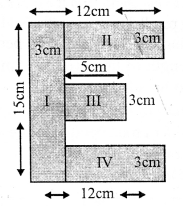and area of rectangle IV = (12 – 3) x 3 = 9 x 3 = 27 cm²
Total area of shaded portion = 45 + 27 + 15 + 27 = 114 cm²

Question 16.
Solution:
Dividing the figure an shown
Area of rectangle I = 3.5 x 0.5 m² = 1.75 m²
Area of rectangle II = (3.5 – 2 x 0.5) x 0.5 = (3.5 – 1) x 0.5 = 2.5 x 0.5 = 1.25 m²
Area of rectangle III = (2.5 – 1) x 0.5 = 1.5 x 0.5 = 0.75 m²
Area of rectangle IV = (1.5 – 1.0) x 0.5 x 0.5 = 0.25 m²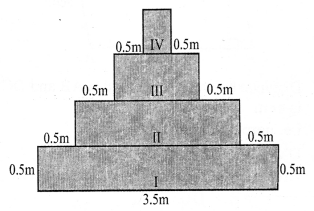Total area of shaded portion = (1.75 + 1.25 + 0.75 + 0.25) m² = 4 m²

Hope given RS Aggarwal Solutions Class 7 Chapter 20 Mensuration Ex 20B are helpful to complete your math homework.

If you have any doubts, please comment below. Learn Insta try to provide online math tutoring for you.